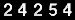# Optimal illumination of a sphere

## Summary

A luminous flux hitting a surface creates a luminance pattern that is dependent on the angle between the target surface and the incoming light. The following problem is discussed and some numerical solutions are presented:
How to arrange the direction vectors of N luminous fluxes of equal intensity illuminating a target sphere with perfectly diffuse reflection to produce a luminance pattern on that sphere that satisfies one of the following 3 criteria
Numerical solutions for N <= 20 are presented including visualizations of the resulting luminance patterns.

## Contents

What's new?  Results sorted by date. (Last update: July 23, 2011).

To give a preliminary idea about luminance distributions, here is an example: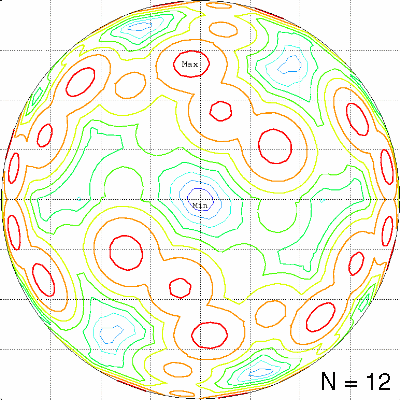The criterion was to minimize the maximum local luminance created by 12 sources. The arrangement of the sources has a mirror symmetry, but there exists a slightly better non-symmetric arrangement. The view shown is perpendicular to the mirror plane. Click on the picture to load a pdf file with a complete set of views for this arrangement. Links to all results are at the end of this page.
If you are just curious and do not want to browse through the details of the problem, I would recommend to view just a few very nice luminance distributions created by symmetric arrangements of light sources. Each configuration is documented in an associated pdf file (size ~300kB) showing the projections of the source locations and luminance patterns on the 6 faces of an enclosing cube:
Minimize maximum for 8 sources, cyclic symmetry order 4,
Maximize minimum for 6 sources, dihedral symmetry order 12,
Minimize difference for 8 sources, dihedral symmetry order 8,
Minimize difference for 13 sources, using modified beam profiles.

## Detailed description of the problem

If we ignore all those features that make "state of the art" ray-traced pictures look "realistic" e.g. specular reflection, ambient lighting, colour dependance etc. then the luminance of an illuminated surface is only determined by the geometric relation of the surface normal vector and the direction of the incoming light.

For a discussion of the terminology see also "Brightness, Luminance and Confusion" by Charles P. Halsted
and the paragraph on "Illumination" (by John Bercovitz) in the "Lens Tutorial".

Please forgive me, if my terminology is inaccurate, or even wrong. Although I studied physics in the early 1970s, light is not my speciality and my native language is not English ;-)

Or even better: help me improving it by sending me a suggestion by E-mail.

For n light sources we have

I = sum over j, j=1..n ( N dot Lj) * Ij
where
N is the surface normal vector,
Lj is the direction unit vector of the incoming light from the jth source
Ij is the intensity of the jth source.

In the following only light sources of equal strength, Ij = 1 are considered.

If the light sources are very far away from the target, then the influence of the source is only determined by its direction vector or more precisely the difference vector between source and target ("directional light") and its intensity.

The most simple object to illuminate is a sphere. It is assumed that illumination of the the target sphere is only by perfectly diffuse reflection. If Lambert's law holds, the viewing angle has no influence on the luminance seen by an observer. Ambient reflection and specular reflection are assumed to be absent.

Here are some examples, created with POVRAY, version 3.0. The current version can be found at POVRAY's web site.

A single light source illuminates one side of the sphere, producing a maximum luminance of 1.0 exactly at its angular location. If the target sphere is located at the origin and has a radius of 1.0, and the direction of the light source is exactly above the north pole (0,0,1), then I = max (0,z).

The following description is from James M. Palmer's "Radiometry and photometry FAQ":
A lambertian sphere illuminated by a distant point source will display a radiance which is maximum at the surface where the local normal coincides with the incoming beam. The radiance will fall off with a cosine dependence to zero at the terminator. If the intensity (integrated radiance over area) is unity when viewing from the source, then the intensity when viewing from the side is 1/p.

Lines of equal luminance are circles of equal latitude (theta=constant in a spherical phi,theta co-ordinate system), as can be seen in the following picture: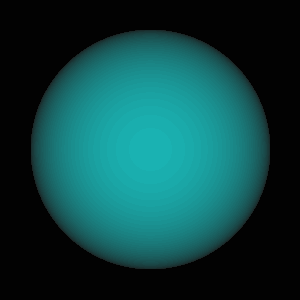If viewed perpendicular to the light direction, the lines of equal luminance appear as straight lines, which looks a little bit surprising: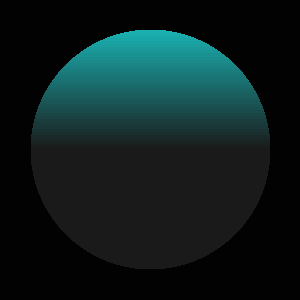The part opposite to the light source remains totally dark (I=0).

If more than one light source is present, a combined luminance pattern is created. In the following pictures

View in the equator plane, perpendicular to the plane of the sources: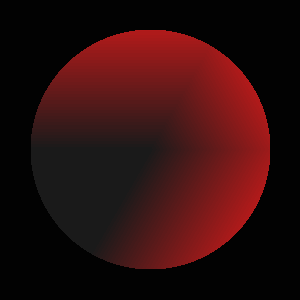two light sources are present, one above the north pole and the other at an angular separation of 120 Degrees in the bottom right corner. In the region of the sphere illuminated by both sources a maximum with I=1 (=2*cos(60Deg)) is created, i.e. the two sources create 3 maxima of equal magnitude (=1).
The following figures show different views of the luminance pattern created by this arrangement.

View in the equator plane, in the plane of the sources: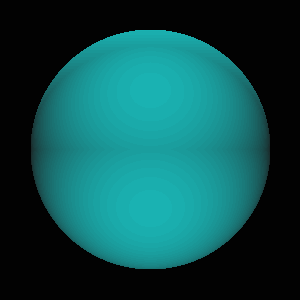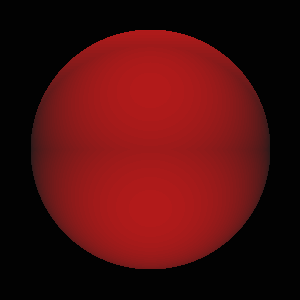View on the north pole: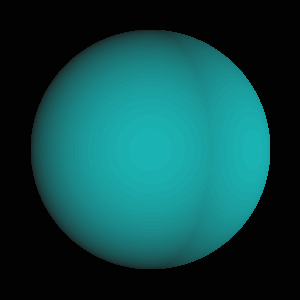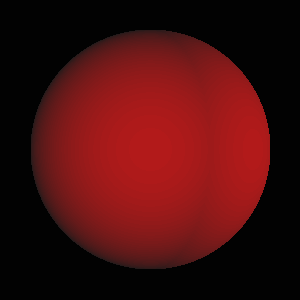In the following picture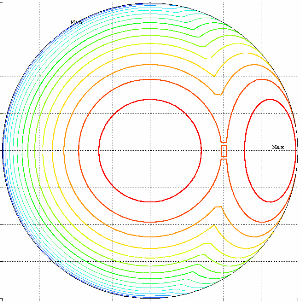lines of equal luminance are represented by colours (red=bright, blue=dark). Those pictures are created by a contour plotting program that uses a grid of triangles and straight line segments to illustrate the luminance distribution (more details see below).

With two and also with three sources, there remains always a region (at least two points for n=3) with I=0.

The "best" configuration for n=2 is putting the sources at opposite sides of the sphere (e.g. above the north and south poles), leaving a dark ring around the equator.

Three sources pose the first, trivial optimization problem: How to arrange the 3 sources to minimize the maximum of luminance over the whole sphere? The only solution is an arrangement on an equilateral triangle, whose plane contains the origin, with arbitrary orientation (e.g. the equator plane). This is the only configuration that avoids mutual angles less than 120 Degrees, which would create a combined intensity maximum >1.0 between two sources.

Starting with four sources, arrangements with I>0 over the whole sphere can be constructed. The most obvious arrangement for 4 sources is the regular tetrahedron. The following figures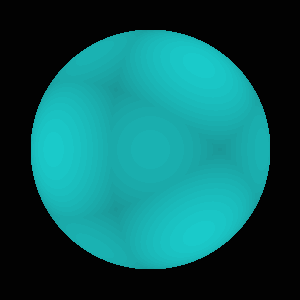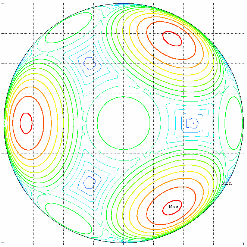show one view of the luminance pattern created by the tetrahedron source arrangement, together with the corresponding contour plot. The input file for POVRAY to produce the first picture is available here.

When looking at this figure, the question arises, if the light sources can be put in some "optimal" arrangement that produces a "uniform" luminance distribution over the whole surface of the sphere.

To describe the "quality" of an arrangement, there are several choices for a target function, 3 different target functions are considered:
1. Maximize the minimum of luminance (make the darkest spot on the sphere as bright as possible)
2. Minimize the maximum of luminance (make the brightest spot on the sphere as dark as possible - the aphotic's solution)
3. Minimize the luminance difference between the brightest and the darkest spot - the social solution)
With a few exceptions for small n (n<6), the optimal configurations are expected to be different for the 3 criteria.

### Here is a somewhat different formulation of the problem:

A point light source far away from a sphere (e.g. a star shining on the earth produces an luminance distribution on the earth's surface that is a function of the relative angle between the surface normal and the direction of the incoming light. The luminance is given by the simple cosinus law. If the light source is arranged exactly above the north pole, the lines of equal luminance are lines of equal latitude. At the equator the luminance has dropped to zero. As the star is invisible, the southern hemisphere will have zero luminance (darkness) from the star above the north pole. If more than one star (light source) is present, the luminance at any point will be the sum of the scalar products of the local surface normal vector with the directions of the incoming contributions from all sources. Only visible sources will contribute, those on the other hemisphere contribute nothing to the local luminance.

The problems are then to arrange the N light sources of equal strength with infinite distance from a target sphere to satisfy the criteria mentioned above.

## A related problem

This class of problems has some relation to inertia confined fusion (ICF) devices, where multiple light beams of high energy lasers are focussed on a small deuterium/tritium target with the aim of compressing the target material to the conditions necessary for fusion reactions. A former version of LLNL's web page about inertial fusion energy had schematic diagrams showing "How inertial fusion works" and on so-called "direct drive" targets that are "uniformly illuminated" from all directions. See the following figure:The physical processes during the energy transfer from the light beams to the target material are rather complicated due to the refractive and absorbtion properties of the plasma surrounding the target. The direct drive approach is now considered as almost infeasible and has been abandoned in favor of the so-called indirect-drive approach.
For a more detailed description see: S.Skupsky, K.Lee: Uniformity of energy deposition for laser driven fusion. J.Appl.Phys. 54 (7), pp.3662-3671, July 1983.
It has been pointed out to me in a private correspondence, that the geometrical part of this problem even has a perfect solution, if one manages to give the laser beams an intensity profile that gives rise to an energy deposition ~cos2(theta) on the target, where theta is the angle relative to the direction of the incoming beam. It is then possible to find arrangements, which produce a constant illumination on the whole target sphere. The conditions for optimal arrangements were first described in the following reference:
A.J. Schmitt: Absolutely Uniform Illumination of Laser Fusion Pellets, NRL Memorandum Report 5221, Naval Research Laboratory Washington D.C., February 17, 1984, also published in Appl. Phys. Lett. 44, pp. 399-401 (1984). The NRL report is available for download.
A similar derivation and some examples consisting of rings of beams with uniform spacing in azimuth and pair-wise opposed beams in rings on the upper and lower hemisphere are given in:
W.W. Simmons: Simple analytic solutions for uniform irradiation of spherical targets. In: First Annual International Conference on Solid State Lasers for Application to Inertial Confinement Fusion, Michel Andre and Howard T. Powell, Editors, Proc. SPIE 2633, pp. 249-254 (1995)

Optimal arrangements with a perfect solution are only possible for N even and >=6.
I have tried to find some good (minimize difference) numerical solutions for odd N, assuming cosine shaped beam profiles and no angular dependence of absorption on the target sphere. The character of the solutions differs from that for constant intensity beams, as can be seen in the following example for N=17: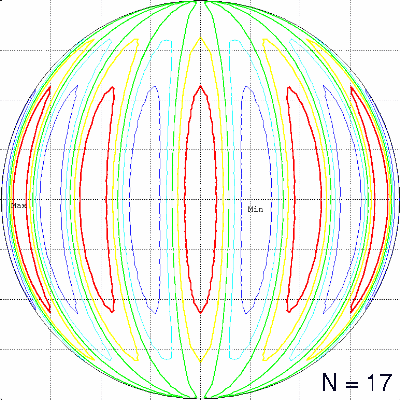Here are my results for N=4 and odd N<=21:

Inertial confinement fusion from Wikipedia.
The Inertial Confinement Fusion Concept.
How ICF works
The US Navy's Nike facility (44+12 beams, designed to shoot at planar targets)
The Nova device, dismantled in 1999, eventually used an arrangement of 2 rings of 5 beams. A picture of the Nova Target Chamber. The National Ignition Facility, which went into operation in 2009, has 192 beams that are focussed on the two ends of a small metal cylinder containing the fusion capsule. The target chamber of NIF also has 24 ports for direct drive experiments.

The Laboratory for Laser Energetics at Rochester, NY operates the 60 beam OMEGA facility specifically tailored for direct drive ICF experiments. A detailed description of OMEGA is found in the National Laser Users' Facility User's Guide, including pictures of the beam configuration and of the radial intensity profiles of the beams (Fig. 2.11), which is indeed not far from the cosine profile mentioned above.

## Optimization problems on the sphere

Some years ago I became interested in optimization problems with simple definition, which were suitable to test numerical methods for global optimization. When looking for examples of difficult optimization problems, especially those with multiple extrema, my attention was caught by the famous placement problems of points on a sphere. Those problems have a very simple definition, but are rather difficult to solve.

For the history of those problems and solutions for low numbers of points the book

L.Fejes Tóth: Lagerungen in der Ebene auf der Kugel und im Raum, Zweite Auflage, Springer-Verlag, Berlin, Heidelberg 1972 (in German)

is still fascinating to read. Also worthwile reading (in German ;-) is the classical article

K. Schütte and B.L. van der Waerden: Auf welcher Kugel haben 5, 6, 7, 8 oder 9 Punkte mit Mindestabstand Eins Platz? Math. Annalen, Bd. 123, pp. 96-124 (1951)

There are at least four well known problems with different criteria, which are described at the Home Page of Neil J.A. Sloane.

1. The packing problem: How should one place n points on a sphere so as to maximize the minimal distance between them? (hostile neighbour problem)
If you are seriously interested in this problem, a "must read" is the article by

Dave A. Kottwitz: The Densest Packing of Equal Circles on a Sphere. Acta Cryst. (1991). A47, pp. 158-165

Most edges of the convex hull of the optimal configurations have the same (minimal) length. I have compiled a table of edge lengths and raytraced pictures showing the distribution of minimal edges for N<=50. Many of the configurations are symmetric; a table with a description of the symmetries (cycles and symmetry group) for N<=50 is available here (Caution: German text :-). An example with coordinates (Phi, Theta, X, Y, Z), list of edges and connectivity is given for N=14. See also: Visualization of this configuration
A list with the orders of the symmetry groups can be found as entryA080865 in Sloane's On-Line Encylopedia of Integer Sequences (OEIS). Due to the missing proofs of optimality for all configurations with N>15 and N not equal 24 this sequence is rather speculative.
For a few values of N (15,62,76,117) the best known solutions are not unique. For a discussion and dynamic visualizations of these configurations see James Buddenhagen's web page.
2. The covering problem: How should one place n points on a sphere so as to minimize the maximal distance of any point on the sphere from the closest one of the n points? This maximum distance is called the covering radius. (covering with equal caps or petrol station placement problem)  Symmetry groups.
3. The maximal volume problem: How should one place n points on a sphere so as to maximize the volume of their convex hull?   Symmetry groups are given in OEIS A081314, raytraced visualizations for n<=21.
4. Arrange N electrically charged particles (e.g. electrons) to minimize the potential energy of the resulting configuration. How should one place n points on a sphere so as to minimize the potential energy Sum 1 / dist(P_i, P_j )?
A description and pictures of "Min-Energy Configurations of Electrons On A Sphere" are provided here.   Symmetry groups.

To my knowledge, the best known solutions of those problems are compiled on the Web Site of Neil Sloane. There are also extensions to higher dimensions than 3. For more information on this topic see the FREQUENTLY-ASKED QUESTIONS ABOUT SPHERES in Dave Rusin's Mathematical Atlas. Anton Sherwood maintains a list of links relating to optimal arrangements of points on a sphere.

If you think, that you have a good method for global optimization, trying to run this method on any of those problems will give you a realistic impression, how hard it can be to find global optima for multidimensional problems - compare your solutions with those listed in Neil Sloane's tables!

The placement problems of N points yields a problem with 2*N-3 variables, since 1 point can be kept fixed, e.g. at the north pole of the sphere, and one angle can be kept fixed for a second point (e.g. phi=0). For the remaining points the angles (phi, theta) in a spherical coordinate system can then be used as optimization variables.

## Numerical procedure

I have used a very robust optimization program (FMINSI, written by myself in Fortran 77, which is based on the Nelder-Mead polytope search method), to search for solutions of this problem, embedded in a strategy for global search. FMINISI is available under the GNU LESSER GENERAL PUBLIC LICENSE.

The problem of finding optimal configurations consists of two coupled global optimization problems. The "inner" problem is to determine the global minimum and/or maximum of I = sum j=1..n (N dot I_j) over all possible orientations of the surface normal vectors of the sphere, which is then used as target function for the source placement problem. As I have found no reliable, elegant technique to find all optima either analytically or by a numerical optimization procedure and I wanted to avoid the rather tricky coupling of two global numerical optimization problems, a brute force approach is used for this problem.

A grid of equally spaced test points (directions) with a prescribed angular separation on the sphere is created and stored. For the global search an angular resolution of 1 Deg is used, creating approximately 41000 test directions, whereas 0.25 Deg resolution is used to produce the final results (approx. 660000 test directions).

Starting at the north pole, circles of equal latitude with the selected spacing are created. On each of this circles equally spaced test points are created. The first circle around the north pole will have 6 test points, whereas the equator circle will produce 360 test points for 1 Deg angular resolution.

Each function evaluation of the numerical optimization requires to evaluate the sum of scalar products of the N light directions with the local surface normal vector (=coordinates of test point on unit sphere), limited to positive contributions (light does not shine through target sphere). This has to be repeated for all test points on the target sphere. The minimum, maximum or difference of both (dependent on the chosen criterion) is then used as the value of the objective function. For an angular separation of alpha [Deg], the number of test points is approximately ((360/alpha)2)/Pi.

Due to the cutoff property (dark rear side) and the contribution of many potential maxima and minima the objective function has a lot of discontinuities in its derivatives, especially around the mimima.

### The optimization strategy

1. Create random configurations of N source directions, using a method described in
George Marsaglia: Choosing a point from the surface of a sphere. The Annals of Mathematical Statistics, 43, pp. 645-646
For those having access to the IMSL library, the corresponding IMSL Stat/Library subroutine for creating random points on the sphere is called RNSPH. Methods to create uniformly-distributed points on a sphere have been discussed in great detail in the sci.math newsgroup. A summary of newsgroup articles by Dave Seaman and by George Marsaglia is available here, including the following description by George Marsaglia.
Generate u and v, uniform in [-1,1], until S=u^2+v^2 < 1,
then return (1-2*S,u*r,v*r), with r=2*sqrt(1-S).
According to Donald E. Knuth, TAOCP Vol.2, 3rd Edition, p.136, this method was first described by Robert E. Knop, CACM 13 (1970), 326.
Typically 100000 random configs are created. The uniform pseudo random numbers needed within the sphere point generator are calculated using the Mersenne Twister.
2. Select the random config that produces the best result for the selected optimization criterion, as starting config for a numerical optimization with a general purpose optimization method and run until convergence. Before starting the optimization, the random config has to be rotated into a standard orientation bringing one point into the north pole and a second point to phi=0. The selected angular separation alpha in steps 1 and 2 is 1 Degree, thus producing approx. 41250 test points.
In the meantime this procedure has been modified; see the corresponding note dated "2001/09/22".
3. Check result configuration for symmetries. I am using an Ada program (available on request) written by my colleague Dr.Walter Möhres. If symmetries are found, use reduced number of variables to describe the arrangement of sources.
4. Run optimization with higher resolution of test points on target sphere, using the results of the previous steps as starting points. Due to the available computer resources, the resolution used for my current results is Alpha=0.25[Deg], which is equivalent to approx. 660000 test points on the target sphere. Sometimes an improvement of the result can be achieved by rotating the whole configuration, bringing another point into the north pole position and restart the optimization.
5. Optional: Rotate optimal configuration into some standard orientation. A preferred choice is to compute the convex hull of the nadirs of the sources on the target sphere and bring a point with a maximal number of meeting edges into the north pole. For symmetric configurations the orientation is either to align an existing symmetry axis of maximal multiplicity in N-S direction or to chose y=0 as the mirror plane.
6. Produce a graphic visualization of the source locations and of luminance distributions on the target sphere.

## Visualization of results

A triangular grid based on a covering of the sphere with icosahedral symmetry, available from N.J.A.Sloane was produced, using ACM algorithm 772 by R.J. Renka.
Reference: Robert J. Renka, "ALGORITHM 772. STRIPACK: Delaunay Triangulation and Voronoi Diagram on the Surface of a Sphere", ACM Trans. Math. Software, Vol. 23, No. 3, Sept. 1997, pp. 416-434.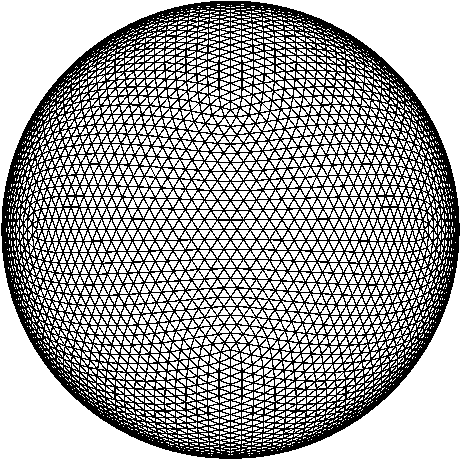The source of the Fortran program is available from NETLIB.
A few more examples of grids created with this method are available here.
The coordinates and the triangle list of the 5072 point mesh are available for download. (One coordinate per line: x1 y1 z1 x2 y2 z2 x3 ...)

The projections of the source locations and of the luminance pattern on the six surfaces of a cube containing the target sphere are plotted and converted into PDF files that can be displayed using the Adobe Reader. Only the visible part of the triangle grid is displayed. The grid consists of 10140 triangles, created on 5072 points on the target sphere. The configuration used was the icosahedral best covering labeled "13,13" in Neil Sloane's "Spherical Codes with Icosahedral Symmetry".

The first heading line indicates the optimization criterion, the second heading shows the numerical value of the achieved optimum. The third line indicates the view.

The plots show scaled luminance values. The scaling used is

Iscal = 100% * 4 * I / N,

which approaches 100% for higher numbers of evenly distributed sources. (A factor of 6 has to be used instead of 4 for the result plots of the laser irradiation problem.) Due to the comparatively coarse resolution of the grid used for plotting, the scaled minimum and maximum values indicated below the contour plot may differ significantly from the intensity value in the header line. This value is computed on a fine grid with 0.25 Degree angular resolution, whereas the scaled values are computed at the nodes of the coarse plotting grid that has only a typical angular resolution of 3 Degrees.

Results are shown for N=4 .. 16 sources. The numerical values of the direction vectors of the sources are stored in ASCII files named XXXXNN.TXT, where XXXX indicates the optimization criterion (MIMA, MAMI, DMMM) and NN indicates the number of sources. Those files have a first line with 4 numbers
• N .. number of sources
• NF .. number of variables used for the final optimization (=2*N-3) for non-symmetric configurations
• I .. the best found result
• CRIT .. indicator for optimization criterion used, i.e.
1. ... maximize minimum
2. ... minimize maximum
3. ... minimize largest difference

The following lines contain the direction vectors of the sources, normalized to length 1. Each line has 6 entries
I PHI THETA X Y Z, where
• I ... number of vector
• PHI ... longitude angle [Degrees]
• THETA ... latitude angle [Degrees], 0 = north pole, 90 = equator, 180 = south pole
• X, Y, Z components of vector, with X2 + Y2 + Z2 = 1.
All location files are part of a single ZIP file, named illumtab.zip.

## Table of best configurations found

For every number of source points 12 plots are collected in one PDF file. To visualize the source locations, a few artificial contour lines are drawn around the "footprint" of every source. The advantage of this technique is, that also sources at the periphery of the projection become visible.

#### Accuracy of the results

The accuracy of the result coordinates is of course limited by the selected angular resolution of the test points on the target sphere, which also limits the accuracy of the achieved optimal intensities. A conservative estimate would be to add sin(Alpha) in the pessimistic direction to the best found result.

Example:

For N=9 sources the numerical optimization result for the "maximize minimum" problem was I=2.1173186, using test points on the target sphere with Alpha=0.25 Deg angular separation. A pessimistic estimate for the true result would be I_true>I_0.25-sin(0.25Deg)=2.1130. From numerical experiments with different values of Alpha in most cases sin(0.5*Alpha) seems to be a more realistic estimate for the difference between the numerical and the true solution giving I_true>2.1151.

#### Comparison of results for different criteria

A diagram comparing the deviations of the best results from absolutely uniform luminance and some remarks on the general character of the solutions for the 3 different criteria is available here.

For viewing a configuration and results, click on the numbers in the table.

### Best configurations found

N Maximize
Minimum
Minimize
Maximum
Minimize
Difference
1 0.0 1.0 1.0
2 0.0 1.0
120 Deg
Separation
1.0
3 0.0 1.0
Isosceles
Triangle
1.0
4 0.8164965
Tetrahedron
1.1547005
Tetrahedron
0.3382040
Tetrahedron
5 0.9396906
Dihedral
Order 8
1.4142137
Dihedral
Order 12
0.5178666
Dihedral
Order 8
6 1.3340707
Dihedral
Order 12
1.6506903
Dihedral
Order 6
0.3438863
Dihedral
Order 12
7 1.5926648
Mirror
Order 2
1.8523984
Cyclic
Order 2
0.2836353
Mirror
Order 2
8 1.8303337
Mirror
Order 2
2.1128430
Dihedral
Order 12
0.3361533
9 2.1174936
Cyclic
Order 3
2.3389220
Cyclic
Order 6
0.2857277
Cyclic
Order 6
10 2.3718607
Cyclic
Order 2
2.5831981
Mirror
Order 2
0.2462976
11 2.6259986 2.8364630 0.2562928
Mirror
Order 2
12 2.9073987
Dihedral
Order 8
3.0849881 0.2080100
Dihedral
Order 8
13 3.1310258 3.3329923 0.2304325
14 3.3844719 3.5890152 0.2314179
15 3.6322515 3.8369949 0.2256613
16 3.8829281 4.0839849 0.2064474
Cyclic
Order 3
17 4.1316900 4.3336964 0.2267036
Mirror
Order 2
18 4.3824286 4.5861354 0.2234712
19 4.6305666 4.8390818 0.2159195
20 4.8798361 5.0889797 0.2225437

Question: Where are results for N>20?
Answer: With increasing N, many solutions exist, which are practically equivalent in the achieved value of the chosen objective function. For the "minimize difference" problem one might choose a desired value for the difference near 0.25 and then run the optimization process until a configuration fulfilling this requirement is found. The asymptotic behavior of the optimal solutions for increasing N is an open question, at least for me. Of course, it is also a question of available computer resources, how far to continue the search. If you have too much idle time available on your 1000-processor MPP machine, send me an E-mail and we can continue searching up to whatever N you want ;-)

## Symmetry of the optimal luminance distributions

Although I did not find a proof for this, the luminance distributions produced on the target sphere by all optimal source configurations seem to have the property

I(x,y,z) = I(-x,-y,-z)

i.e. the intensities at antipode locations are equal (for N>1).
This property is also found for the non-symmetric source configurations.

The numerical solutions for the illumination problem (minimize difference between maximum and minimum luminance on target sphere) with beams having a cosine shaped radial intensity profile indicate the following conjecture:
Optimal solutions of this problem fulfill

I(x,y,z) + I(-x,-y,-z) = N/3

In other words: The sum of energy densities at antipodal points is constant.
My currently best numerical solution for N=11 does not fulfill this criterion. If I should fail to find a better solution, then the conjecture has to be limited to N>11.

My most recent results indicate, that there exists a systematic way to construct very good (maybe the best possible) solutions for odd N>=15: Arrange the maximum possible odd number of beams with equal angular distance in an equatorial ring. The remaining even number of beams is split into 2 rings with antipodal orientation near the poles. For N=15 and N=17 the two pole rings consist of 3 beams each, for N=19,21 of 4 beams ... The necessary number of beams in the pole rings is determined by the intensity created by the equator ring at the equator that has to be balanced at the pole locations.
Example: Equatorial rings of 9 or 11 beams need pole rings of 3 beams, 13 and 15 equator beams need 4-rings, ..., 49 and 51 equator beams need 13-rings.

## A note on symmetry and "obvious" results

Nearly everybody asked for an intuitive solution of the sphere illumination problems would probably guess that highly symmetric configurations are optimal or give at least solutions close to the optimum. It is quite surprising, that none of the highly symmetric arrangements fulfills this expectation with the exception of N=4, where the regular tetrahedron is indeed the best possible solution for all 3 different criteria.
Here is a comparison for the arrangements corresponding to the vertices of the regular polyhedra (Platonic solids) with my best solutions for N=6,8,12,20. For viewing the configuration, click on the name in the first column.

### Comparison with Platonic solids

Platonic
Solid
N I_min best I_max best Diff_mm best
Octahedron 6 1.000 1.334 1.732 1.651 0.732 0.344
Cube 8 1.633 1.830 2.309 2.113 0.676 0.336
Icosahedron 12 2.753 2.907 3.236 3.085 0.483 0.208
Dodekahed. 20 4.780 4.880 5.236 5.089 0.456 0.228

Even the perfectly symmetric arrangement with icosahedral symmetry looses against the non-symmetric optimal configurations, when applied to the illumination problems discussed here. Nevertheless, the optimal results have symmetries for low numbers of sources:

Known optimal symmetric solutions:

Maximize minimum: N<=10 and N=12
Minimize maximum: N<=10
Minimize difference: N<=7, N=9, N=11 and N=12; N=14 is very close to mirror symmetry, but the symmetric solution is not stable.

The kind of symmetry and the order of the symmetry group of the source arrangements are indicated in the XXXXBEST.TXT files that are part of "illumtab.zip".

## Some sub-optimal symmetric configurations

There are also some symmetric configurations, which give results close to my best non-symmetric solutions. The source positions of some examples are compiled in a file illoktab.zip, with the naming convention XXXXNNLn.TXT, where XXXXNN is analoguous to the naming of the result files for the best configurations, L indicates local optimum, and n is a counter. Some comments on recent updates to this list are available here.

For viewing the configuration, click on the value in the last column.

### Symmetric arrangements

N Criterion Symmetry Order
I
7 Maximize
Minimum
Cyclic 3 1.5832877
7 Maximize
Minimum
Dihedral 4 1.5616652
7 Minimize
Difference
Cyclic 3 0.2855948
8 Maximize
Minimum
Dihedral 16 1.825501
8 Maximize
Minimum
Dihedral 8 1.8199916
8 Minimize
Maximum
Cyclic 4 2.1139913
8 Minimize
Difference
Dihedral 8 0.4140986
8 Minimize
Difference
Dihedral 4 0.3475444
9 Maximize
Minimum
Dihedral 6 2.1173196
9 Minimize
Maximum
Cyclic 2 2.3468831
9 Minimize
Difference
Mirror 2 0.2976573
9 Minimize
Difference
Cyclic 3 0.2916300
10 Maximize
Minimum
Mirror 2 2.3474007
10 Minimize
Maximum
Cyclic 3 2.5876093
10 Minimize
Difference
Cyclic 3 0.2511883
10 Minimize
Difference
Mirror 2 0.2642903
10 Minimize
Difference
Mirror 2 0.2647603
11 Maximize
Minimum
Dihedral 4 2.5977113
11 Minimize
Maximum
Cyclic 6 2.8445046
11 Minimize
Maximum
Cyclic 2 2.8387887
11 Minimize
Difference
Mirror 2 0.2886286
12 Maximize
Minimum
Cyclic 2 2.8377018
12 Maximize
Minimum
Dihedral 6 2.8915510
12 Minimize
Maximum
Mirror 2 3.0882597
12 Minimize
Difference
Mirror 2 0.2684853
12 Minimize
Difference
Cyclic 3 0.2297292
13 Maximize
Minimum
Mirror 2 3.1238143
13 Maximize
Minimum
Mirror 2 3.1231952
14 Minimize
Maximum
Mirror 2 3.5918217
18 Minimize
Difference
Mirror 2 0.2386804

This page has been updated on September 13, 2014 by Hugo Pfoertner.
Comments and suggestions are always welcome.

##### Back to Contents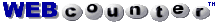says you are visitor